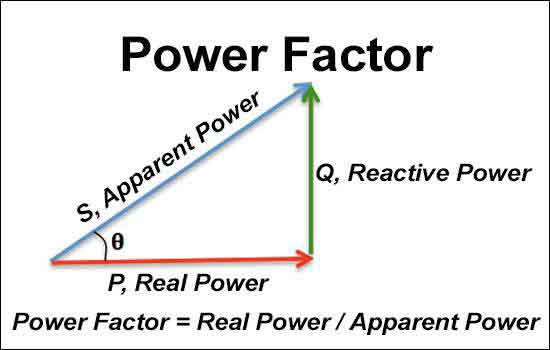# Power Factor Formula - Understanding AC Circuits

#### By R.W. Hurst, EditorThe power factor formula is a vital parameter for understanding the efficiency of an electrical circuit. It helps to determine the power factor (PF) of a circuit, which is the ratio of the actual power to the apparent power. A low PF can result in inefficiencies in the circuit and increased energy bills. PF correction is a method used to improve the PF of a circuit, and the power triangle can represent the relationship between the PF, active power, and reactive power.

PF is a crucial parameter that determines the efficiency and quality of an electrical circuit. In AC circuits, the PF represents the ratio of true power to apparent power. The PF formula helps understand the relationship between active, reactive, and apparent power.

In this article, we will delve into the details of the PF formula, its significance in electrical circuits, and how to measure it.

What is Power Factor in AC Circuit?

PF is the ratio of the actual power (P) consumed by a load to the apparent power (S) supplied. For example, in an AC circuit, the apparent power is the product of the voltage and current flowing through it. On the other hand, the actual power or active power is the power that is consumed by the load and used to perform work.

Formula:

The PF formula is given as follows:

Power Factor (PF) = P/S

Where P is the actual power and S is the apparent power. The PF is a dimensionless quantity and is always between 0 and 1.

In an electrical circuit, the PF indicates how efficiently the circuit uses the supplied power. A high PF indicates that the circuit is using power efficiently, while a low PF indicates that the circuit is not using power efficiently.

What is Power Factor?

The PF is the cosine of the phase angle (θ) between an AC circuit's voltage and current waveforms. The phase angle represents the time delay between the voltage and current signals. For example, the voltage and current signals are in-phase in an ideal circuit with resistive loads, and the PF is 1. In this case, the apparent and actual power is the same.

However, the voltage and current signals are not in phase in circuits with inductive or capacitive loads. This results in a phase difference between the voltage and current waveforms, leading to a lagging or leading PF.

The PF is an essential parameter for determining the efficiency of an electrical circuit. A low PF indicates that the circuit is not using power efficiently and is, therefore, inefficient.

How do You Find the Power Factor?

To find a PF of a wer, you need to measure the circuit's apparent power (S) and the actual power (P). The apparent power is the product of the circuit's RMS voltage (V) and RMS current (I). The actual power can be measured using a wattmeter or by using the following formula:

P = V x I x PF

Once you have measured the actual power and apparent power, you can use the formula to determine the PF.

Example:

Suppose you have a circuit with an RMS voltage of 120 V and an RMS current of 5 A. You measure the actual power as 500 W. To find the PF, you can use the following formula:

Apparent Power (S) = V x I
S = 120 V x 5 A = 600 VA

Power Factor (PF) = P/S
PF = 500 W/600 VA = 0.83

Therefore, the PF of the circuit is 0.83.

What Does a Power Factor of 0.8 Mean?

A PF of 0.8 means the circuit is not using power efficiently. In this case, the circuit uses only 80% of the supplied power to perform useful work. The remaining 20% of the power is lost as reactive power.

How do You Determine Power Factor from kWh?

To determine the PF from kWh, you need to know the circuit's apparent power (S) and the active energy consumed (kWh). The formula to arrive at PF from kWh is as follows:

PF = kWh / (S x h)

where kWh is the active energy consumed in kWh, S is the apparent power, and h is the number of hours.

How do You Determine 3-Phase Power Factor?

In a 3-phase electrical circuit, the PF can be arrived at using the following formula:

PF = P / (3 x V x I x Cos(θ))

where P is the actual power, V is the line voltage, I is the line current, and Cos(θ) is the PF angle.

In a 3-phase circuit, the PF can be lagging, leading, or unity. A lagging PF occurs in circuits with inductive loads, while a leading PF occurs in circuits with capacitive loads.

Power Factor Correction:

A poor PF can cause several issues in an electrical circuit, including increased power consumption, reduced efficiency, and higher energy bills. PF  correction is a method used to improve the power factor of a circuit.

The most common PF correction method is adding capacitors to the electrical circuit. Capacitors can store and release energy, which helps to offset the reactive power and improve the PF.

Power Triangle:

The PF, active power, and reactive power can be represented geometrically using a power triangle. The power triangle represents the relationship between these parameters in an electrical circuit.

• 1
• 2
• 3
• 4
• 5
• 6
• 7
• 8
• 9
• 10
• 11
• 12
• 13
• 14
• 15
• 16
• 17
• 18
• 19
• 20
• 21
• 22
• 23
• 24
• 25
• 26
• 27
• 28
• 29
• 30
• 31

Top